#### Did you know plotnine as the grammar of graphics for Python?

Plotnine is the implementation of the R package ggplot2 in Python. It replicates the syntax of R package ggplot2 and visualizes the data with the concept of the grammar of graphics. It creates a visualization based on the abstraction of layers. When we are making a bar plot, we will build the background layer, then the main layer of the bar plot, the layer that contains title and subtitle, and etc. It is like when we are working with Adobe Photoshop. The plotnine package is built on top of Matplotlib and interacts well with Pandas. If you are familiar with the ggplot2, it can be your choice to hand-on with plotnine. The template of ggplot2 is as follows.

`ggplot(data = <DATA>) +   <GEOM_FUNCTION>(     mapping = aes(<MAPPINGS>),     stat = <STAT>,      position = <POSITION>  ) +  <COORDINATE_FUNCTION> +  <FACET_FUNCTION>`

Simple explanation:

• ggplot(data = <DATA>): create a background layer. We set the dataset as the input for visualization
• <GEOM_FUNCTION>(): our main layer to create a certain data visualization. For instance, if we are going to create a bar plot, <GEOM_FUNCTION> will be geom_bar, etc
• <COORDINATE_FUNCTION>: a layer to set up both horizontal and vertical axis, title, subtitle, the element of the axis, etc.
• <FACET_FUNCTION>: an optional layer to split the plots into a matrix of panels

It’s important to know well about the scale measurement from the data.

#### Let’s hands-on with the Olympics data

To discuss and practise with the plotnine package, we are using the Olympics data 1896–2016. It’s a cleaned dataset but has several columns with different scale measurement, so theoretically we can create a lot of data visualization and insights from the data.

You can directly download the data from the dropbox HERE. The file contains two datasets, athlete event and noc region. The athlete event is a record of all athlete, their characteristics, health information and citizenship, and medal acquisition from 1896–2016. Whereas, the noc region records the National Olympic Committee (NOC).

`# Dataframe manipulationimport pandas as pd`
`# Linear algebraimport numpy as np`
`# Data visualization with matplotlibimport matplotlib.pyplot as plt# Use the theme of ggplotplt.style.use('ggplot')`
`# Data visualization with plotninefrom plotnine import *import plotnine`
`# Set the figure size of matplotlibplt.figure(figsize = (6.4,4.8))`

Our first task, of course, to import the data into our Jupyter Notebook and explore the values from columns. The athlete event dataset has 271,116 records or rows and 15 columns or attributes.

`athlete_data = pd.read_csv('datasets/athlete_events.csv')print('Dimension of athlete data:\n{}'.format(len(athlete_data)),      'rows and {}'.format(len(athlete_data.columns)),'columns')athlete_data.head()`

Columns description of athlete event data:

• ID: unique ID or identifier for the athlete
• Name: the name of the athlete
• Sex: the gender of the athlete
• Age: the age of an athlete in a certain year
• Height: athletes weight (in centimetres)
• Weight: athletes weight (in kilogram)
• Team: the country of athletes
• NOC: the National Olympic Committee (must be merged with the noc region data to get the region name)
• Games: the Olympics competition event
• Year: the time when the competition was held
• Season: it has two unique value, winter and summer
• City: city or region where the competition was held
• Sport: a sport that athletes participate in
• Event: the competition event programme
• Medal: medal acquisition by the athlete. It includes Gold, Silver, and Bronze. The NaN means the athlete didn’t win the competition or medal

Our second data is the noc region. It has 230 records or rows and 3 columns or attributes. The NaN in the notes column means that they are not recorded.

`regions_data = pd.read_csv('datasets/noc_regions.csv')print('Dimension of region data:\n{}'.format(len(regions_data)),      'rows and {}'.format(len(regions_data.columns)),'columns')regions_data.head()`

After exploring the raw dataset, it’s time to merge those into one cleaned data to visualize. We conduct the left join with the athlete event as the left table and NOC column as the index level names to join on. It produces the cleaned data with 271,116 rows and 17 columns.

`full_data = athlete_data.merge(regions_data,on='NOC',how='left')print('Dimension of full data:\n{}'.format(len(full_data)),      'rows and {}'.format(len(full_data.columns)),'columns')full_data.head()`

#### Let’s create data viz using the Olympics data

Basically, there are a lot of data visualization types, such as bar plot, histogram, time series plot, pie chart, etc. You can easily find out the catalogue of data visualization HERE. In this tutorial, we will create 8 types of data viz using plotnine package.

#### 1 Histogram using plotnine

A histogram is the most commonly used graph to show frequency distributions. It lets us discover and show the underlying frequency distribution of a set of numerical data. To construct a histogram from numerical data, we first need to split the data into intervals, called bins.

`# Create a histogram(    ggplot(data = full_data[full_data['Age'].isna() == False])+    geom_histogram(aes(x = 'Age'),                   fill = '#c22d6d',                   bins = 20)+  # Set number of bin    labs(title = 'Histogram of Athlete Age',         subtitle = '1896 - 2016')+    xlab('Age')+    ylab('Frequency')+    theme_bw())`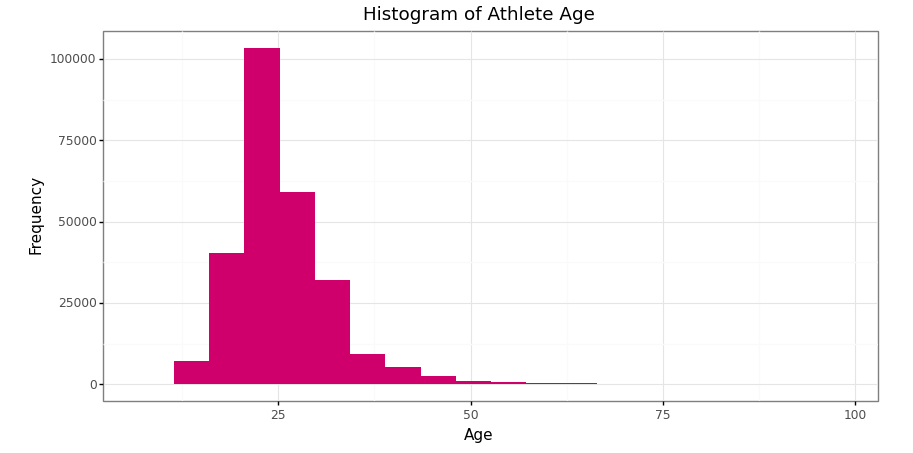Histogram of athlete’s age in Olympics data 1896–2016 (Image by Author)

#### 2 Area chart using plotnine

An area chart is an extension of a line graph, where the area under the line is filled in. While a line graph measures change between points, an area chart emphasizes the data volume.

`# Data manipulation before making time series plot# 1 Each country medals every yearmedal_noc = pd.crosstab([full_data['Year'], full_data['NOC']], full_data['Medal'], margins = True).reset_index()# Remove index namemedal_noc.columns.name = None# Remove last row for total column attributemedal_noc = medal_noc.drop([medal_noc.shape - 1], axis = 0)medal_noc`
`# 2 General championmedal_noc_year = medal_noc.loc[medal_noc.groupby('Year')['All'].idxmax()].sort_values('Year')medal_noc_year`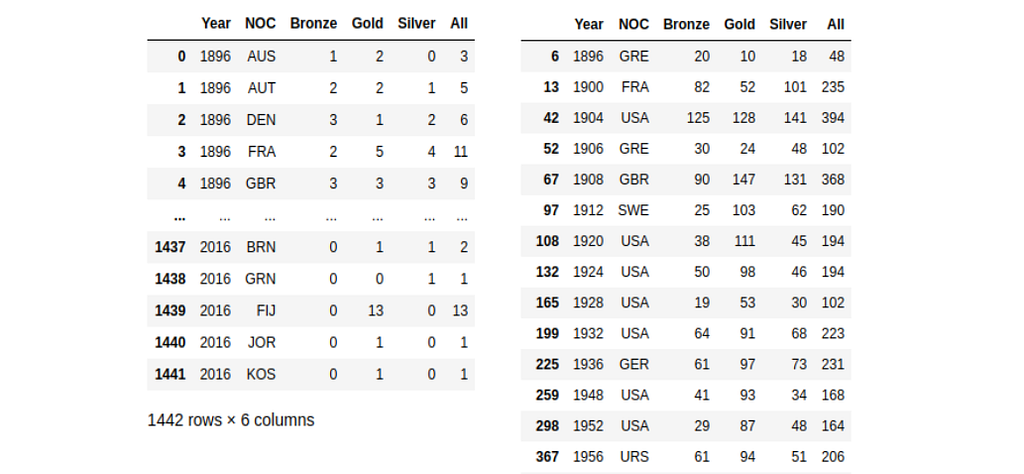Medals acquisition by countries in 1896–2016 (left) and medals acquisition by the general winner in 1896–2016 (right) (Image by Author)
`# Create a time series plot(    ggplot(data = medal_noc_year)+    geom_area(aes(x = 'Year',                  y = 'Gold',                  group = 1),              size = 1,              fill = '#FFD700',              alpha = 0.7)+    geom_area(aes(x = 'Year',                  y = 'Silver',                  group = 1),              size = 1,              fill = '#C0C0C0',              alpha = 0.8)+    geom_area(aes(x = 'Year',                  y = 'Bronze',                  group = 1),              size = 1,              fill = '#cd7f32',              alpha = 0.8)+    scale_x_discrete(breaks = range(1890,2020,10))+    labs(title = 'Area Chart of Medals Acquisition',         subtitle = '1896 - 2016')+    xlab('Year')+    ylab('Frequency')+    theme_bw())`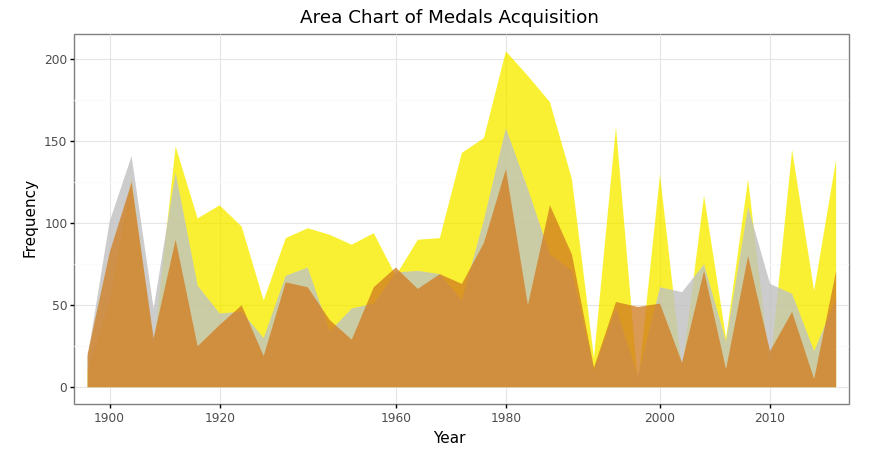Area chart of total medals acquisition in 1896–2016 in Olympics data (Image by Author)

#### 3 Bar plot using plotnine

Bar plot has a similar aim to the histogram. It lets us discover and show the underlying frequency distribution of a set of categorical data. As we know that categorical data can not be measured by the mathematics equation, such as multiplication, subtraction, etc but can be counted.

`# Data manipulation before making bar plot# The country that won the most olympics - tablemedal_noc_count = pd.DataFrame(medal_noc_year['NOC'].value_counts()).reset_index()medal_noc_count.columns = ['NOC','Count']medal_noc_count`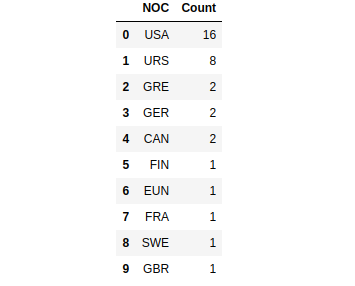Top ten countries top that won the most Olympics competition 1896–2016 (Image by Author)
`# Create a bar plot(    ggplot(data = medal_noc_count)+    geom_bar(aes(x = 'NOC',                 y = 'Count'),             fill = np.where(medal_noc_count['NOC'] == 'USA', '#c22d6d', '#80797c'),             stat = 'identity')+    geom_text(aes(x = 'NOC',                  y = 'Count',                  label = 'Count'),              nudge_y = 0.7)+    labs(title = 'Bar plot of Countries that Won Olympics',         subtitle = '1896 - 2016')+    xlab('Country')+    ylab('Frequency')+    scale_x_discrete(limits = medal_noc_count['NOC'].tolist())+    theme_bw()    )`
Note: we are able to using geom_label as the alternative of geom_text. It has a similar argument too. Please, try by yourself!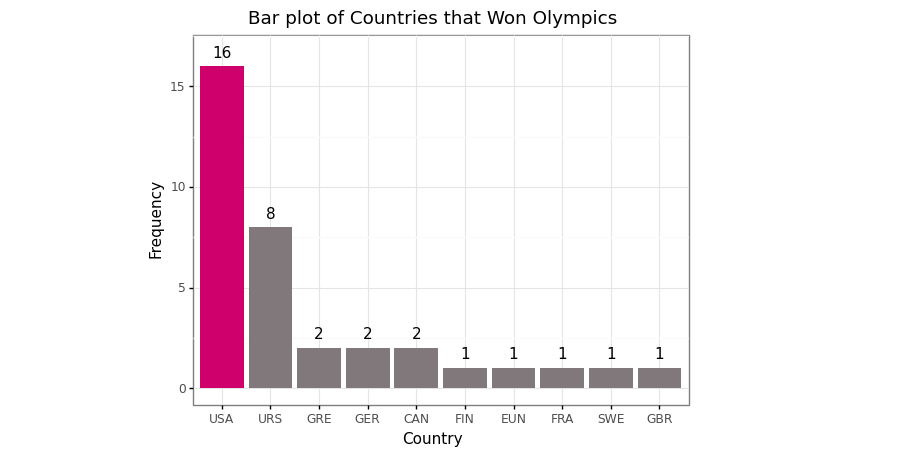Bar plot of the top ten countries top that won the most Olympics competition 1896–2016 (Image by Author)
`# Data manipulation before making bar plot# Top five sport of USA# 1 Cross tabulation of medalsmedal_sport = pd.crosstab([full_data['Year'], full_data['NOC'], full_data['Sport']], full_data['Medal'], margins=True).drop(index='All', axis=0).reset_index()medal_sport`
`# 2 Cross tabulation of medals in sportsmedal_sport_usa = medal_sport[medal_sport['NOC'] == 'USA']medal_sport_usa_count = medal_sport_usa.groupby('Sport')['All'].count().reset_index()medal_sport_usa_count_10 = medal_sport_usa_count.sort_values('All', ascending=False).head(10)medal_sport_usa_count_10`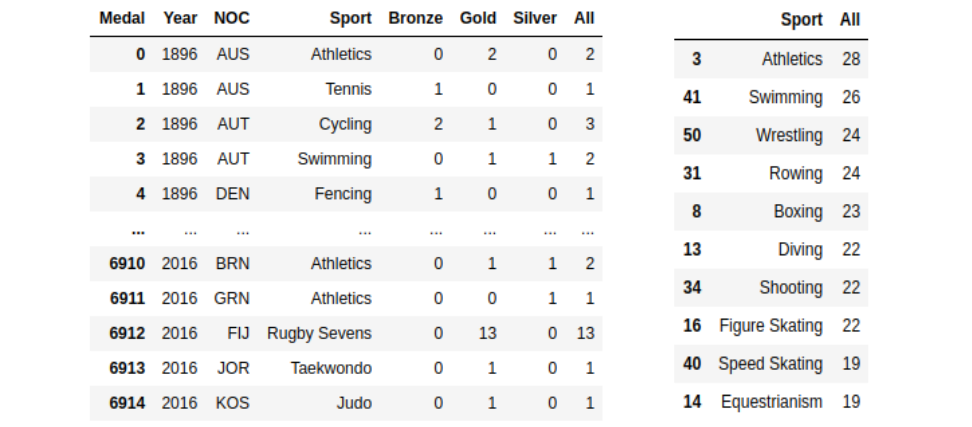Number of medals each sport won by country 1896–2016 (left) and number of medals each sport won by USA 1896–2016 (right) (Image by Author)
`# Create a bar plot(    ggplot(data = medal_sport_usa_count_10)+    geom_bar(aes(x = 'Sport',                 y = 'All',                 width = 0.6),             fill = np.where(medal_sport_usa_count_10['Sport'] == 'Figure Skating', '#c22d6d', '#80797c'),             stat = 'identity')+    geom_text(aes(x = 'Sport',                  y = 'All',                  label = 'All'),              nudge_y = 0.9)+    labs(title = 'Bar plot of Top Ten Sport Won by USA',         subtitle = '1896 - 2016')+    xlab('Sport')+    ylab('Frequency')+  scale_x_discrete(limits = medal_sport_usa_count_10['Sport'].tolist()[::-1])+    theme_bw()+    coord_flip())`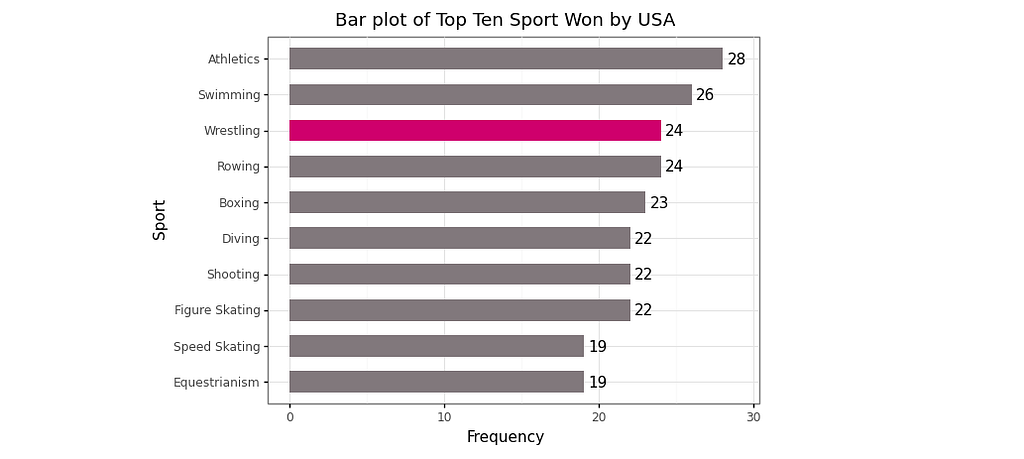Bar plot of top ten sports won by USA 1896–2016 (Image by Author)

#### 4 Box and Whisker plot using plotnine

Box and Whisker plot is a standardized way of displaying the distribution of data based on a five-number summary:

• Minimum value
• The first quartile (Q1)
• Median
• The third quartile (Q3)
• Maximum value

We need to have information on the dispersion of the data. A box and Whisker plot is a graph that gives us a good indication of how the values in the data are spread out. Although box plots may seem primitive in comparison to a histogram or density plot, they have the advantage of taking up less space, which is useful when comparing distributions between many groups or data.

`# Data manipulationdata_usa_urs = full_data[full_data['NOC'].isin(['USA','URS'])]data_usa_urs = data_usa_urs[data_usa_urs['Age'].isna() == False].reset_index(drop = True)`
`# Create a box plot(    ggplot(data = data_usa_urs)+    geom_boxplot(aes(x = 'NOC',                     y = 'Age'),                     fill = '#c22d6d',                 show_legend = False)+    labs(title = 'Box and Whisker plot of Age',         subtitle = '1896 - 2016')+    xlab('Country')+    ylab('Age')+    coord_flip()+    theme_bw())`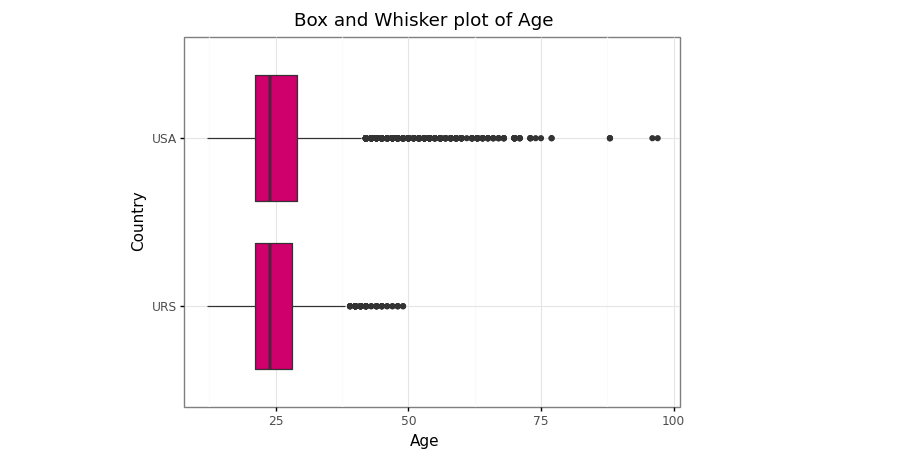Box and Whisker plot of age distribution between USA and URS in 1896–2016 (Image by Author)

#### 5 Pie chart using plotnine

Pie charts are very popular for showing a compact overview of a composition or comparison. It enables the audience to see a data comparison at a glance to make an immediate analysis or to understand information quickly. While they can be harder to read than column charts, they remain a popular choice for small datasets.

Note: we can’t a pie chart via plotnine package because unfortunately, the function coord_polar which is needed to created pie chart is not in the plotnine API
`# Data manipulation before making pie chart# Dominant season# 1 Select the majority season each yeardata_season_year = pd.crosstab(full_data['Year'], full_data['Season']).reset_index()data_season_year.columns.name = Nonedata_season_year['Status'] = ['Summer' if data_season_year.loc[i,'Summer'] > data_season_year.loc[i,'Winter'] else 'Winter' for i in range(len(data_season_year))]data_season_year`
`# 2 Dominant season each yeardominant_season = data_season_year.groupby('Status')['Year'].count().reset_index()dominant_season`
`# Customize colors and other settingscolors = ['#c22d6d','#80797c']explode = (0.1,0) # Explode 1st slice# Create a pie chartplt.pie(dominant_season['Year'], explode = explode, labels = dominant_season['Status'], colors = colors, autopct = '%1.1f%%', shadow = False, startangle = 140)plt.title('Piechart of Dominant Season') # Titleplt.axis('equal')plt.show()`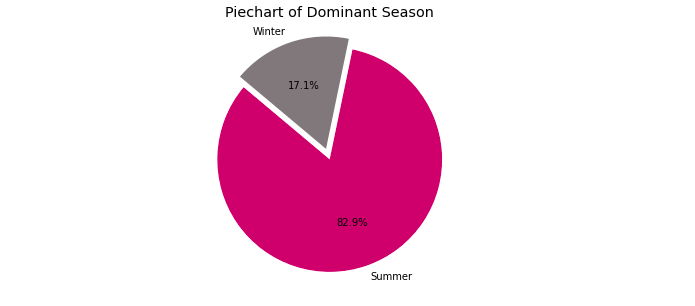Pie chart of the majority season since Olympics event 1896–2016 (Image by Author)

#### 6 Time series plot using plotnine

A time series plot is a plot that shows observations against time. According to the Chegg Study, the uses of the time-series plot are listed.

• Time series plot easily identifies the trends.
• Data for long periods of time can be easily displayed graphically
• Easy future prediction based on the pattern
• Very useful in the field of business, statistics, science etc
`# Data manipulation before making time series plotleft = medal_noc_year[medal_noc_year['NOC'] == 'USA']right = data_season_yeardata_season_usa = left.merge(right, on='Year', how='left')data_season_usa`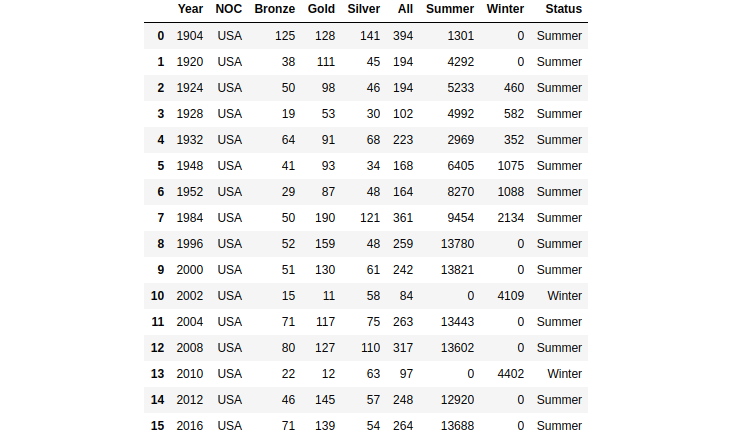The medal acquisition of USA and majority season in Olympics event 1904–2016 (Image by Author)
`# Create a time series plot(    ggplot(data = data_season_usa)+    geom_line(aes(x = 'Year',                  y = 'All',                  group = 1),              size = 1.5,              color = '#c22d6d')+    geom_point(aes(x = 'Year',                   y = 'All',                   group = 1),               size = 3,               color = '#000000')+    geom_text(aes(x = 'Year',                  y = 'All',                  label = 'All'),              nudge_x = 0.35,              nudge_y = 10)+    scale_x_discrete(breaks = range(1900,2020,10))+    labs(title = 'Line Chart of Medals Acquisition (USA)',         subtitle = '1896 - 2016')+    xlab('Year')+    ylab('Frequency')+    theme_bw())`Time series plot of medals acquisition of USA in Olympics event 1904–2016 (Image by Author)

#### 7 Scatter plot using plotnine

A scatterplot is a type of data visualization that shows the relationship between two numerical data. Each point of the data gets plotted as a point whose (x, y) coordinates relates to its values for the two variables. The strength of the correlation can be determined by how closely packed the points are to each other on the graph. Points that end up far outside the general cluster of points are known as outliers.

`# Data manipulation before making scatter plot# 1 Select the majority season each yeardata_medals = full_data[full_data['Medal'].notna()]left = data_medals[(data_medals['NOC'] == 'USA') & (data_medals['Medal'].notna())].groupby('Year')['Sport'].nunique().reset_index()right = medal_noc[medal_noc['NOC'] == 'USA']sport_medal_usa = left.merge(right, on = 'Year', how = 'left')sport_medal_usa`
`corr_sport_all = np.corrcoef(sport_medal_usa['Sport'], sport_medal_usa['All'])[0,1]`
`# Print statusprint('Pearson correlation between number of sport and total of medals is {}'.format(round(corr_sport_all,3)))`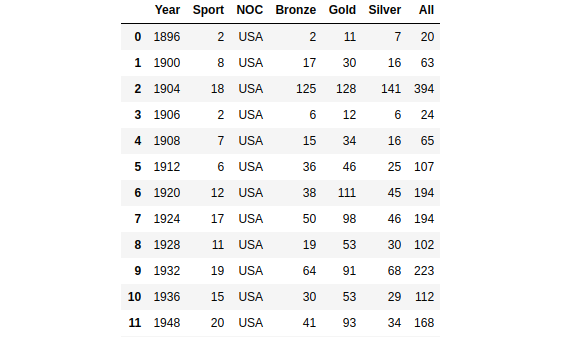The medal acquisition of USA and number of sports in Olympics event 1896–2016 (Image by Author)
`# Create a scatter plot(    ggplot(data = sport_medal_usa)+    geom_point(aes(x = sport_medal_usa['Sport'],                   y = sport_medal_usa['All'],                   size = sport_medal_usa['All']),               fill = '#c22d6d',               color = '#c22d6d',               show_legend = True)+    labs(title = 'Scatterplot Number of Sport and Total of Medals',         subtitle = '1896 - 2016')+    xlab('Number of Sport')+    ylab('Total of Medals')+    theme_bw())`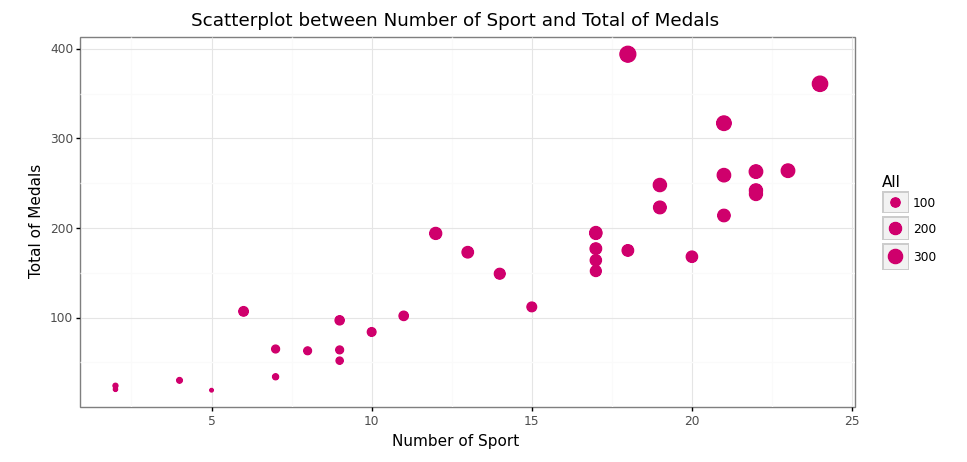Scatter plot between the number of sports and total medals acquisition (Image by Author)

#### 8 Facet wrapping using plotnine

According to the plotnine official site, facet_wrap() creates a collection of plots (facets), where each plot is differentiated by the faceting variable. These plots are wrapped into a certain number of columns or rows as specified by the user.

`# Data manipulation before making box and whisker plotdata_usa_urs['Medal'] = data_usa_urs['Medal'].astype('category')data_usa_urs['Medal'] = data_usa_urs['Medal'].cat.reorder_categories(['Gold', 'Silver', 'Bronze'])data_usa_urs`

The pre-processing is done, now let’s create a visualization!

`# Create a box and whisker plot(    ggplot(data = data_usa_urs[data_usa_urs['Medal'].isna() == False])+    geom_boxplot(aes(x = 'NOC',                     y = 'Age'),                 fill = '#c22d6d')+    labs(title = 'Box and Whisker plot of Age',         subtitle = '1896 - 2016')+    xlab('Country')+    ylab('Age')+    theme_bw()+    facet_grid('. ~ Medal'))`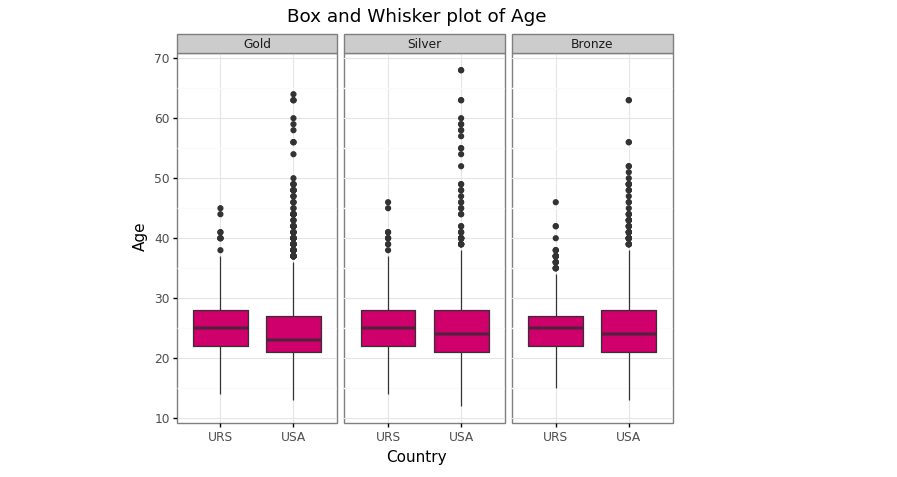Box and Whisker plot of age between USA and URS by medals type (Image by Author)

#### Conclusion

The plotnine package is a wonderful data viz package in Python. It replicates the ggplot2 package in R and the user can easily create a visualization more beautiful. It accommodates all the ggplot2 package, but for several viz like a pie chart, it doesn't support yet! This is not the problem because we can use the matplotlib as another alternative.

#### References

 Anonim. Making Plots With plotnine (aka ggplot), 2018. https://monashdatafluency.github.io/.

 J. Burchell. Making beautiful boxplots using plotnine in Python, 2020. https://t-redactyl.io/.

 S. Prabhakaran. Top 50 ggplot2 Visualizations — The Master List (With Full R Code), 2017. http://r-statistics.co/.Introduction to Plotnine as the Alternative of Data Visualization Package in Python was originally published in Towards Data Science on Medium, where people are continuing the conversation by highlighting and responding to this story.